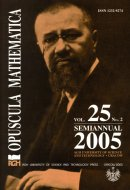Opuscula Math. 25, no. 2 (2005), 169-179

Opuscula Mathematica

# Calculation of distribution of temperature in three-dimensional solid changing its shape during the process

Bogusław Bożek
Czesław Mączka

Abstract. The present paper suplements and continues [Bożek B., Filipek R., Holly K., Mączka C.: Distribution of temperature in three-dimensional solids. Opuscula Mathematica 20 (2000), 27-40]. Galerkin method for the Fourier–Kirchhoff equation in the case when $$\Omega(t)$$ – equation domain, dependending on time $$t$$, is constructed. For special case $$\Omega(t) \subset \mathbb{R}^2$$ the computer program for above method is written. Binaries and sources of this program are available on http://wms.mat.agh.edu.pl/~bozek.

Keywords: parabolic partial differential equations, non-stationary distribution of heat, finite element method, Galerkin method.

Mathematics Subject Classification: 65M60, 65Y99.

Full text (pdf)

• Bogusław Bożek
• AGH University of Science and Technology, Faculty of Applied Mathematics, al. Mickiewicza 30, 30-059 Cracow, Poland
• Czesław Mączka
• AGH University of Science and Technology, Faculty of Applied Mathematics, al. Mickiewicza 30, 30-059 Cracow, Poland